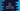# C++ program to Print all even numbers from 1 to 100## C++ program to Print all even numbers from 1 to 100:

In this post, we will learn how to print all even numbers from 1 to 100 in C++. We will learn different ways to do that. With this program, you will learn how to use loops and how to print content in C++.

A number is called even if it is properly divisible by 2, i.e. if we divide this number by 2, the reminder will be 0.

## Method 1: By using a for loop:

Let’s try first by using a for loop. Below is the complete program that prints all even numbers from 1 to 100 using a for loop:

``````#include <iostream>
using namespace std;

int main()
{
for (int i = 1; i <= 100; i++)
{
if (i % 2 == 0)
{
cout << i << endl;
}
}

return 0;
}``````

Here,

• We are using a for loop that runs from i = 1 to i = 100.
• Inside the loop, we are checking if the current value of i is properly divisible by 2 or not. Modulo operator % returns the remainder value. So, if we use number % 2, it will return the reminder if we divide number by 2. So, if it is 0, it means that that number is an even number.

If you run this program, it will print all the even numbers from 1 to 100 as like below:

``````2
4
6
8
10
12
14
16
18
20
22
24
26
28
30
32
34
36
38
40
42
44
46
48
50
52
54
56
58
60
62
64
66
68
70
72
74
76
78
80
82
84
86
88
90
92
94
96
98
100``````

## Method 2: By using a for loop and without using modulo operator:

We have another way to solve this problem. We can use the for loop and without any modulo operator. The loop will start from i = 2 and it will end in i = 100. But after each step, we will increment the value of i by 2.

``````#include <iostream>
using namespace std;

int main()
{
for (int i = 2; i <= 100; i = i + 2)
{
cout << i << endl;
}

return 0;
}``````

It will print the same output.

It will be faster than the previous example because we are running the loop only for even numbers, not for all numbers.

## Method 3: By using a while loop:

Similar to a for loop, we can use a while loop as well. It works in a similar way. while loop keeps running and checks for a condition on each iteration. Once this condition becomes false, it stops.

Below is the complete program:

``````#include <iostream>
using namespace std;

int main()
{
int i = 2;
while (i <= 100)
{
cout << i << endl;
i = i + 2;
}

return 0;
}``````

It works in a similar way.

• We have initialized one variable i as 2 before the loop starts.
• The while loop runs till the value of i is less than or equal to 100.
• Inside the loop, we are printing the value of i and also we are updating it as i = i+2. So, it will point to the next even number.

If you run this program, it will print the same output.